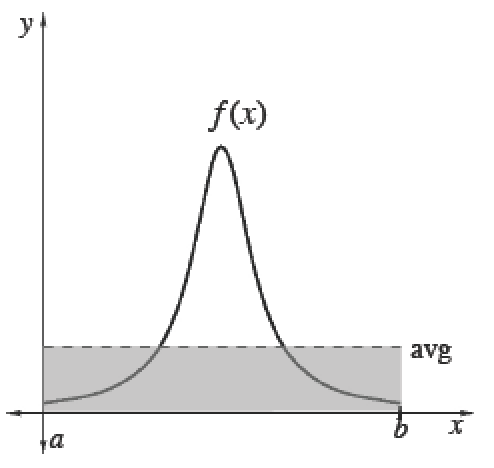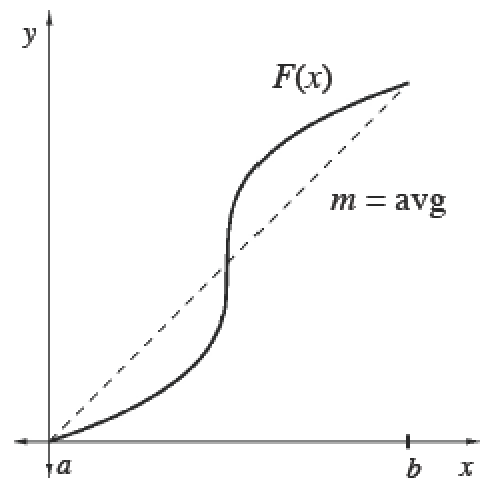### Home > APCALC > Chapter 6 > Lesson 6.4.3 > Problem6-154

6-154.

For each part below, calculate the average value of $g(t)$ over the given interval. You may solve analytically or use your graphing calculator to evaluate.

1. $g(t) = 2t^2 – 3$ over $[0, 4]$

2. $g(t) = 3e^t + \ln(t + 1)$ over $[0, 1]$

Examine the Math Notes box about about Mean (average) Value. Notice that there are two strategies to compute Mean (average) Value.

Strategy 1: Compute the average area under the curve.
Strategy 2: Compute a slope of a secant line.

WARNING: These strategies can NOT be used interchangeably. The strategy you choose depends on the function you are given:
$f(x)$ or $F(x)$.

And it depends on the question you are being asked:
Find average y-value? or Find average rate of change?

Average (Mean) Values

 To calculate the mean (average) value of a finite set of items, add up the values of items and divide by the number of items.Integrals help us add over a continuous interval. Therefore, for any continuous function $f$ :$\frac{\int_a^bf(x)dx}{b-a}=$ mean value of $f$ over $[a, b]$Since $\int _ { a } ^ { b } f ( x ) d x$, we can also calculate the average value of any function $f$ using its antiderivative $F$. Its average slope gives the average rate of change of $F$, which is the same as the average value of $f.$$\frac{\int_a^bf(x)dx}{b-a}=\frac { F ( b ) - F ( a ) } { b - a }=$  mean rate of change of $F ′$ over $[a, b]$